# Bihar Board 12th Maths Model Question Paper 4 in English Medium

Bihar Board 12th Maths Model Papers

## Bihar Board 12th Maths Model Question Paper 4 in English Medium

Time : 3 Hours 15 Min
Full Marks: 100

Instructions for the candidates :

1. Candidates are required to give their answers in their own words as far as practicable.
2. Figure in the right-hand margin indicates full marks.
3. While answering the questions, the candidate should adhere to the word limit as far as practicable.
4. 15 Minutes of extra time has been allotted for the candidate to read the questions carefully.
5. This question paper is divided into two sections. Section-A and Section-B
6. In Section A, there are 1-50 objective type questions which are compulsory, each carrying 1 mark. Darken the circle with blue/black ball pen against the correct option on the OMR Sheet provided to you. Do not use Whitener/Liquid/ Blade/Nail on OMR Sheet otherwise result will be invalid.
7. In section-B, there are 25 short answer type questions (each carrying 2 marks), out of which only 15 (fifteen) questions are to be answered.
A part from this there is 08 Long Answer Type questions (each carrying 5 marks), out of which 4 questions are to be answered.
8. Use of any electronic device is prohibited.

Objective Type Questions

There are 1 to 50 objective type questions with 4 options, choose the correct option which is to be answered on OMR Sheet (50 × 1 = 50)

Question 1.
What type of relation on the set A = {1,2,3,4} given by R = {(1,3), (4,2), (2,4), (2,3), (3,1)} is
(a) Reflexive
(b) Transitive
(c) Symmetric
(d) None of these
(d) None of theseQuestion 2.
If the operation * defined as (a * b) = a2 + b2 then the value of (3 * 4) * 5 is
(a) 650
(b) 125
(c) 625
(d) 3125
(a) 650

Question 3.
If f: R → R defined as f(x) = 3x. Then the function is
(a) one-one into
(b) one-one onto
(c) Manyone into
(d) Many one onto
(b) one-one onto

Question 4.
$$\tan ^{-1} \frac{2 x}{1-x^{2}}=$$
(a) 2sin-1x
(b) sin-12x
(c) tan-12x
(d) 2tan-1x
(d) 2tan-1xQuestion 5.
The value of $$\cos ^{-1}\left(-\frac{1}{2}\right)$$ is
(a) $$\frac{\pi}{3}$$
(b) $$\frac{\pi}{6}$$
(c) $$\frac{2 \pi}{3}$$
(d) $$\frac{3 \pi}{4}$$
(c) $$\frac{2 \pi}{3}$$

Question 6.
tan-1x + cot-1x = :
(a) π
(b) $$\frac{\pi}{2}$$
(c) $$\frac{2 \pi}{3}$$
(d) $$\frac{3 \pi}{4}$$
(b) $$\frac{\pi}{2}$$

Question 7.
$$\left[\begin{array}{ccc} b c & 1 & 1 / a \\ c a & 1 & 1 / b \\ a b & 1 & 1 / c \end{array}\right]=?$$
(a) 1/abc
(b) 0
(c) abc
(d) None of these
(b) 0

Question 8.
$$\left|\begin{array}{cc} \cos 15^{\circ} & \sin 15^{\circ} \\ \sin 75^{\circ} & \cos 75^{\circ} \end{array}\right|=?$$
(a) 1
(b) π/2
(c) 0
(d) None of these
(c) 0Question 9.
If λ ∈ R and ∆ = $$\left|\begin{array}{ll} \mathbf{a} & \mathbf{b} \\ \mathbf{c} & \mathbf{d} \end{array}\right|$$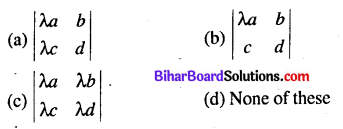(c) $$\left|\begin{array}{ll} \lambda a & \lambda b \\ \lambda c & \lambda d \end{array}\right|$$

Question 10.
If A = $$\left[\begin{array}{lll} 1 & 2 & 3 \\ 4 & 5 & 6 \end{array}\right]$$ Then which of following is A’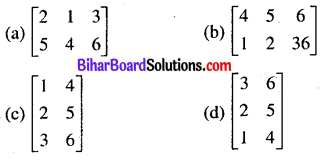(c) $$\left[\begin{array}{ll} 1 & 4 \\ 2 & 5 \\ 3 & 6 \end{array}\right]$$

Question 11.
If y = sin (log x) then $$\frac{\mathrm{dy}}{\mathrm{dx}}=?$$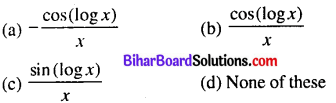$$\frac{\cos (\log x)}{x}$$

Question 12.
If y = x3 + 4x + 6 then $$\frac{d^{2} \cdot y}{d x^{2}}=?$$
(a) 3x3 + 4
(b) 3x2
(c) 6x
(d) 3x
(c) 6xQuestion 13.
If y = log√x then the value of $$\frac{d y}{d x}$$ is(a) $$\frac{1}{2 x}$$

Question 14.
If y = sin2x then the value of $$\frac{d^{2} y}{d x^{2}}$$ is
(a) 2 sin 4x
(b) -4 sin 2x
(c) 4 sin 2A
(d) 4 cos2 2x
(b) -4 sin 2x

Question 15.
The ratio of rate of change x2 and log x is
(a) 2x
(b) 2x2
(c) 2
(d) 2/3
(b) 2x2

Question 16.
If $$y=\sec ^{-1}\left[\frac{\sqrt{x}+1}{\sqrt{x}-1}\right]+\sin ^{-1}\left[\frac{\sqrt{x}-1}{\sqrt{x}+1}\right]$$ then $$\frac{d y}{d x}$$ = :
(a) 1
(b) π
(c) π/2
(d) 0
(d) 0

Question 17.
The vectors a1î + a2 ĵ + a3k̂ and b1î + b2 ĵ + b3 ĵ perpendicular to each other if
(a) $$\frac{a_{1}}{b_{1}}=\frac{a_{2}}{b_{2}}=\frac{a_{3}}{a_{3}}$$
(b) a1b1 + a2b2 + a3b3
(c) a1b2 + b2a1 + a3b2 = 0
(d) None of these
(b) a1b1 + a2b2 + a3b3
Question 18.
k̂ × k̂ =
(a) 0
(b) 1
(c) |k|2
(d) None of these
(a) 0

Question 19.
The scalar product of the vectors 5î + yĵ – 3k̂ and 3î – 4ĵ + 7k̂is
(a) 10
(b) -10
(c) 15
(d) -15
(b) -10Question 20.
The vector equation of the line which passes through the points A(3,4, -7) and B(l, -1,6) is(a) $$\vec{r}=(3 \hat{i}+4 \hat{j}-7 \hat{k})+\lambda(\hat{i}-\hat{j}+6 \hat{k})$$

Question 21.
For what value of x, î + ĵ + k̂ is a unit vector ?(b) $$\frac{1}{\sqrt{3}}$$

Question 22.
Distance between the planes r̂. n̂ = p1 and r̂. n̂ = p2 is
(a) p1 – p2
(b) |p1-p2|
(c) $$\frac{\left|p_{1}-p_{1}\right|}{2}$$
(d) None of these
b) |p1-p2|

Question 23.
$$\int_{0}^{2 / 3} \frac{d x}{4+9 x^{2}}$$ is equal to
(a) $$\frac{\pi}{6}$$
(b) $$\frac{\pi}{12}$$
(c) $$\frac{\pi}{24}$$
(d) $$\frac{\pi}{4}$$
(c) $$\frac{\pi}{24}$$

Question 24.
$$\int_{0}^{\pi / 2} \frac{\sin x}{\sin x+\cos x} d x$$ dx is equal to
(a) $$\frac{\pi}{2}$$
(b) $$\frac{\pi}{4}$$
(c) π
(d) None of these
(b) $$\frac{\pi}{4}$$Question 25.
$$\int x^{2} e^{x^{3}} d x=$$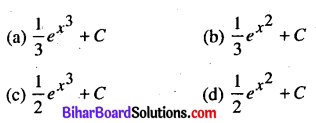(a) $$\frac{1}{3} e^{x^{3}}+C$$

Question 26.
The value of $$\int_{0}^{\pi / 4} \sin 2 x d x$$ is
(a) 1
(b) 1/2
(c) 1/3
(d) 1/4
(b) 1/2

Question 27.
Area lying in the first quadrant and bounded by the circle x2 + y2 = 4 and x = 0, x = 2 is
(a) π
(b) $$\frac{\pi}{2}$$
(c) $$\frac{\pi}{3}$$
(d) $$\frac{\pi}{4}$$
(a) π

Question 28.
The order of differentied equation
$$\left(\frac{d^{2} y}{d x^{2}}\right)+2\left(\frac{d y}{d x}\right)^{3}+9 y=0$$ is
(a) 2
(b) 3
(c) 4
(d) None of these
(a) 2

Question 29.
The differential equation of the family of straight line which passes through the origin is(b) $$y \frac{d y}{d x}=x$$

Question 30.
The solution of $$\frac{d y}{d x}$$ = 1 + x + y + xy is
(a) x – y = k(1 + xy)
(b) log(1 + y)=x + $$\frac{x^{2}}{2}$$ + k
(c) log (1 + x) = y + $$\frac{y^{2}}{2}$$ + k
(d) None of these
(b) log(1 + y)=x + $$\frac{x^{2}}{2}$$ + kQuestion 31.
If $$\vec{a}=2 \vec{i}-5 \vec{\jmath}+\vec{k}$$ and $$\vec{b}=4 \vec{i}+2 \vec{\jmath}+\vec{k}$$ then $$\vec{a} \cdot \vec{b}=:$$
(a) 0
(b) -1
(c) 1
(d) 2
(b) -1

Question 32.
If $$2 \vec{i}+\vec{j}+\vec{k}, 6 \vec{i}-\vec{j}+2 \vec{k}$$ and $$14 \vec{i}-5 \vec{\jmath}+4 \vec{k}$$ are the position vectors of the point A, B, C respectively, then
(a) A, B, C are collinear
(b) A, B, C are non-collinear
(c) $$\overrightarrow{A B} \perp \overrightarrow{B C}$$
(d) None of these
(a) A, B, C are collinear

Question 33.
If $$|\vec{a} \times \vec{b}|=|\vec{a} \cdot \vec{b}|$$ then angle between $$\vec{a}$$ and $$\vec{b}$$ is n
(a) 0
(b) $$\frac{\pi}{2}$$
(c) $$\frac{\pi}{4}$$
(d) π
(c) $$\frac{\pi}{4}$$

Question 34.
Angle between the vectors 2î + 3ĵ + 2k̂ and î + 4ĵ + 5k̂ is
(a) 30°
(b) 90°
(c) 45°
(d) 60°
(b) 90°

Question 35.
l, m, n and l1 m1 n1 are the direction cosines of two lines are perpendicular to each other if: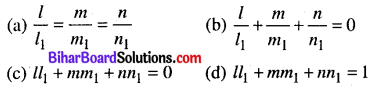(c) ll1 + mm1 + nn1 = 0

Question 36.
A straight line passes through (2, -1,3) and its direction ratio’s are 3, -1,2. Then the equation of the line is(b) $$\frac{x-2}{3}=\frac{y+1}{-1}=\frac{z-3}{2}$$

Question 37.
The direction ratio’s of the normal to the plane 7x + 4y – 2z + 5 = 0 is
(a) (7,4,5)
(b) (7,4,-2)
(c) (7,4,2)
(d) (0,0,0)
(b) (7,4,-2)Question 38.
Angle between the lines $$\vec{r}=(4 \hat{i}-\hat{j})+s(2 \hat{i}+\hat{j}-3 k)$$ and
$$\vec{r}=(\hat{i}-\hat{j}+2 \hat{k})+t(\hat{i}-3 \hat{j}+2 \hat{k})[latex] is 3n n 2n n (a) [latex]\frac{3 \pi}{2}$$
(b) $$\frac{\pi}{3}$$
(c) $$\frac{2 \pi}{3}$$
(d) $$\frac{\pi}{6}$$
(b) $$\frac{\pi}{3}$$

Question 39.
The vectors î + ĵ and ĵ + k̂ are perpendicular then unit vectors is equal to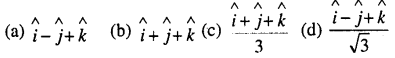(c) \begin{aligned} &\wedge \wedge \quad \wedge\\ &\frac{i+j+k}{3} \end{aligned}

Question 40.
Which of the following is value of constant c of Rolle’s theorem when fix) = 2x3 – 5x2 – 4x + 3, x ∈$$\left[\frac{1}{3}, 3\right]$$
(a) $$-\frac{1}{3}$$
(b) $$\frac{2}{3}$$
(c) -2
(d) 2
(d) 2

Question 41.
The modulus of the vector $$2 \vec{i}-7 \vec{j}-3 \vec{k}$$ is
(a) √61
(b) √62
(c) √64
(d) √32
(b) √62

Question 42.
The projection of the vector î – 2ĵ + k̂ on 2î – ĵ + k̂ is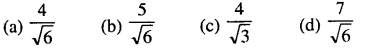(b) $$\frac{5}{\sqrt{6}}$$

Question 43.
If $$|\vec{a}|=\sqrt{26}|\vec{b}|=7$$ and $$|\vec{a} \times \vec{b}|=35$$ then $$\vec{a} \cdot \vec{b}=$$
(a) 8
(b) 7
(c) 9
(d) 12
(b) 7

Question 44.
The function Z = ax + by in Linear programming problem is called
(a) objective Function
(b) constraints
(c) Feasible solution
(d) corner point
(a) objective Function

Question 45.
If P(A) = $$\frac{1}{2}$$ ,P(B) = 0 then P$$\frac{A}{B}$$ is
(a) 0
(b) 1/2
(c) 1
(d) Not defined
(d) Not definedQuestion 46.
If P( A) = 0.8, P(B) = 0.5 and P(B/A) = 0.4 then P(A∪B) equal to :
(a) 0.78
(b) 0.88
(c) 0.98
(d) 1.08
(c) 0.98

Question 47.
Two events A and B are mutually independent if
(a) P(A’ B’) = [1 – P(A)][1 – P(B)]
(b) P(A) = P(B)
(c) P(A) + P(B) = 1
(d) A and B are muttually exclusive
(a) P(A’ B’) = [1 – P(A)][1 – P(B)]

Question 48.
The probability of getting doublet with one die is
(a) $$\frac{2}{3}$$
(b) $$\frac{1}{6}$$
(c) $$\frac{5}{6}$$
(d) $$\frac{5}{36}$$
(b) $$\frac{1}{6}$$

Question 49.
If A and B are two events such that if P(A∪B) = P(a) Then
(a) P(A/B)= 1
(b) P(B/A)= 1
(c) P(A/B) = 0
(d) P(A/B) = 0
(d) P(A/B) = 0

Question 50.
If A and B are imitualy exclusive events, then
(a) P (A/B) = 0
(b) P (B/A) = 0
(c) P(A∪B) = 0
(d) P (A ∩ B) = 0
(d) P (A ∩ B) = 0Non-Objective Type Questions

Q. No. 1 to 25 are of Short Answer type carry of 2 marks each. Answer any 15 questions. (15 × 2 = 30)

Question 1.
Let a function f: R→R defined as $$f(x)=\frac{x^{2}}{x^{2}+1}$$ ∀ x ∈ R . Then find f-1.
Let f: R→R $$defined as f(x)=\frac{x^{2}}{x^{2}+1}$$
Taking 1, 1, ∈ R, we get, Clearly f(1) = f(-1) = 1/2
So, f is manyone function. Thus f-1 is not exists.

Question 2.
If $$\tan ^{-1}\left(\frac{1-x}{1+x}\right)=\frac{1}{2} \tan ^{-1} x$$ , then find x.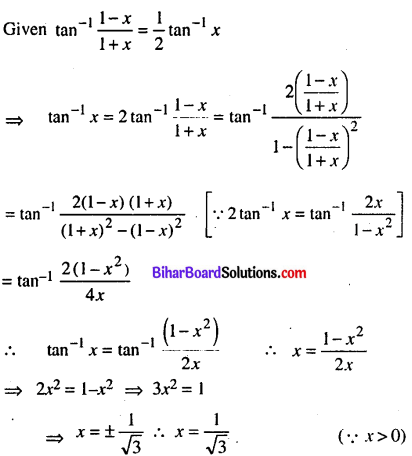Question 3.
Applying properties of determinant, prove that:
$$\left|\begin{array}{lll} 1 & 1 & 1 \\ a & b & c \\ b c & c a & a b \end{array}\right|=(a-b)(b-c)(c-a)$$Question 4.
If $$\left[\begin{array}{cc} a-b & 2 a+c \\ 2 a-b & 3 c+a \end{array}\right]=\left[\begin{array}{cc} -1 & 5 \\ 0 & 13 \end{array}\right]$$ then find a,b,c and d
$$\left[\begin{array}{cc} a-b & 2 a+c \\ 2 a-b & 3 c+a \end{array}\right]=\left[\begin{array}{cc} -1 & 5 \\ 0 & 13 \end{array}\right]$$
a – b = -1 …………(1)
2a – b = 0 …………(2)
2a + c = 5…….(3)
3c + d = 13……(4)
Subtract (1) from (2), we get
2a – b – a + b = 0 + 1 ⇒ a = 1
Frorn (2), 2a – b = 0 ⇒ 2.1 – b ⇒ b = 2
Putting the value ofa in(3), we get 2a + c = 5 ⇒ 2.1 +c = 5
⇒ c = 5 – 2=3 , Lastly putting the value of c in (4), we get
3c + d = 13 ⇒ 3 x 3 + d = 13 ⇒ d= 13 – 9 = 4
Thus a= 1,b=2,c=3and d=4

Question 5.
If y = ex(sin x + cos x) then prove that
$$\frac{d^{2} y}{d x^{2}}-2 \frac{d y}{d x}+2 y=0$$ we haveQuestion 6.
Verify the continuity at x = 0 of the function f(x)=\left\{\begin{aligned} \frac{|\sin x|}{x}, & x \neq 0 \\ 1, & x=0 \end{aligned}\right.Question 7.
Differentiate: sec {tan ( √x)} with respect to x.Question 8.
Find the projection of the vector î + ĵ + k̂) in the direction ĵQuestion 9.
Show that the tangents to the curve y = 7x3 +11 at the points where x = 2 and x= 2 are parallel.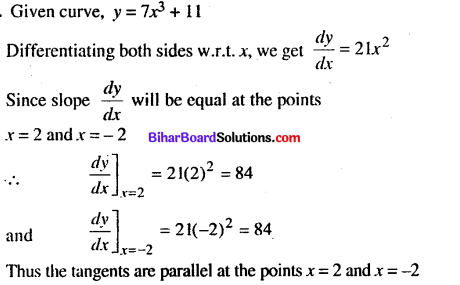Question 10.
Integrate: $$\int \frac{(\log x)^{2}}{x} d x$$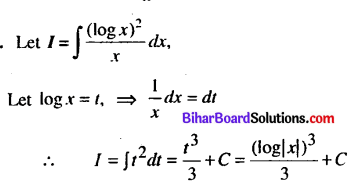Question 11.
Find the value of $$\int_{0}^{\pi / 4} \tan ^{2} x d x$$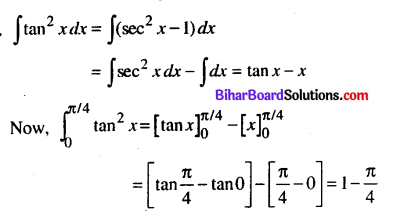Question 12.
Solve the differential equation $$\frac{d y}{d x}$$ = 1 + x + y + xy
We have, $$\frac{d y}{d x}$$ = (1 + x) + y(1 + x) = (1 + x)(1 + y)
$$\int \frac{d y}{1+y}$$ = (1 + x)dx = ∫dx + ∫ xdx
log(1 + y) = x + $$\frac{x^{2}}{2}+c$$

Question 13.
Form the differential equation of the family of circles toucingthey-axis at Origin
The equation of circle of radius a and centre (a, 0) and which passes through the origin with y-axis is
(x – a)2 + y2 = a2
or x2 + y2 = 2ax …(1)
Differentiating w.r.t. x, we get
2x + 2yy’= 2a
or, x + yy’ = a ……(2)Putting the value of a from (2) in (1), we get
x2 + y2 = 2x(x + yy’) = 2x2 + 2 xy y’
or $$2 x y \frac{d y}{d x}+x^{2}-y^{2}=0$$
Which is the required differential equation

Question 14.
Show that the lines $$\vec{r}=(\hat{i}+\hat{j}-\hat{k})+\lambda(3 \hat{i}-\hat{j})$$ and
$$\vec{r}=(4 \hat{i}-\hat{k})+\mu(2 \hat{i}+3 \hat{k})$$ intersect each other. Find their point of intersection.
Since the lines intersects for the any value of λ and μ the refore the value of $$\vec{r}$$ is equal for them
Then (î + ĵ – k̂) + λ(3î – ĵ) =(4î – k̂) + µ(2î+3k)
⇒ (î + 3λ)î+(1 – λ)ĵ – k̂= (4 + 2µ)î + (3µ – 1)k̂)
⇒ 1 + 3λ = 4 + 2µ, 1 – λ = 0 and 3µ – 1 = -1
⇒ 3λ – 2µ = 3 …………(1)
λ = 1 ………….. (2)
and µ = 0 …(3)
clearly, λ = 1 and µ = O are sastis fled
Putting λ = 1. we get r̂ = (î + ĵ – k̂) + λ(3î – ĵ)
r̂ = (4î + 0ĵ – k̂)
Thus point of intersection of line is (4, 0, -1)Question 15.
Find the equation of the through the point (5, -2, 4) and which is parallell to (2î – ĵ + 3k̂)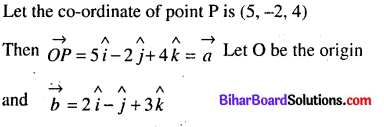Question 16.
What is the maximum value of the function sin x + cos x
Let the interval be [0, 2π] is given
Then f(x) = sin A’ + cos x
f'(x) = cos x – sin x
For maximum and minimum value,f'(A) = 0Question 17.
Prove that : $$\overrightarrow{(\vec{a}-\vec{b})} \times(\vec{a}+\vec{b})=2(\vec{a} \times \vec{b})$$Question 18.
Solve : $$\frac{d y}{d x}+2 y \tan x=\sin x, y=0$$ when x = π/3
We have $$\frac{d y}{d x}$$ + 2ytan x = sin x
This is linear differential equation, where P = 2tanx and Q = sin.xQuestion 19.
If A and B are independent events, then prove that A and B’ are independent events.
Given, A and B are independent then
P(A∩B) = P(A).P(B)
Now, A = (A∩B) ∪ (A∩B’)
⇒ P(A) = P(A∩B) + P(A∩B’)
P(A) = P(A).P(B) +P(A∩B’)
⇒ P(A∩B’) = P(A)-P(A) P(B)
⇒ P(A∩B’) = P(A) [ 1 -P(B) ]
⇒ P(A∩B’)= P(A).P(B’)
Thus A and B’ are independent.

Question 20.
If $$|\overrightarrow{\mathbf{a}}+\overrightarrow{\mathbf{b}}|=|\overrightarrow{\mathbf{a}}-\overrightarrow{\mathbf{b}}|$$ the prove that $$\overrightarrow{\mathbf{a}} \perp \overrightarrow{\mathbf{b}}$$Question 21.
Solve the differential equation $$\frac{d y}{d x}$$= 1 – x + y – xyQuestion 22.
If P(A) = 0.8, P(B) = 0.5 and P(B/A) =0.4, then find
P(A ∩ B)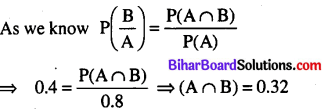Question 23.
Show that the relation R in the set Z of integers given by R = {(a, b): 3 divides a – b} is an equivalence relation.
Let a,b,c ∈ Z
(i) (a, a) ∈ R ⇒ aRa ⇒ a – a = O ∈ Z, divisible by 3 since 0∈Z, which is divisible by 3, so R is reflexive relation.
(ii) (a, b) ∈ R ⇒ (b, a) ∈ R ⇒ (a – b) is divisible by ⇒ (b – a) is divisible by 3. Since (a – b) is divisible by 3 so (b- a) is also divisible by 3, ∀ a, b ∈ Z. so R is symmetric relation.
(iii) (a, b) ∈ R, (b, c) ∈ R ⇒ (a, c) ∈ R ⇒ (a – b) is divisible by 3, (b – c) is divisible by 3
⇒ a – b + b – c = (a – c) is divisible by 3.
Since (a – b) is divisible by 3, (b – c) is divisible by 3
⇒ (a – c) is divisible by 3, ∀ a, b, c ∈ Z. SO R is transitive relation.
Since R is reflexsive, symmetric and transitive relations. Therefore R is an equivalence relation.

Question 24.
Solve tan-12x + tan -13x = π/4or, 5x = 1 – 6x2
or, 6x2+ 5x – 1 = 0
or, 6x2 + 6x – x – 1 = 0
or, 6x (x + 1) – 1(x+ 1) = 0 – or,
(6x- 1)(x+ 1) = 0
∴ 6x – 1 = 0
or x + 1 = 0
i.e, x = 1/6 or, x = -1
Since x ≠ -1 so x = 1/6Question 25.
Find X and Y if X + Y =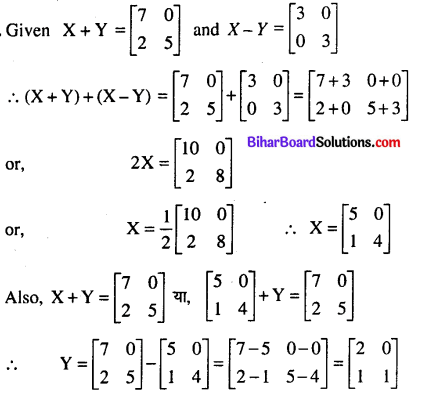Questions No. 26 to 33 are Long Answer Type Questions. Each question carry 5 marks. Answer any 4 question. (4 × 5 = 20)

Question 26.
Show that semi-vertical angle of right circular cone of given surface are anf maximum value is sin -1(1/3)
Let r be the radius, Z be sland height and h be height of the cone. Then s = πr2 + πrl
or $$l=\frac{s-\pi r^{2}}{\pi r}$$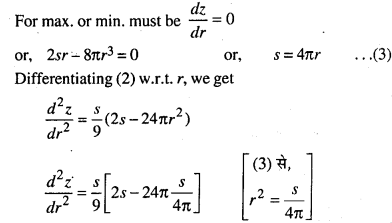Question 27.
Prove that the function sinx(1 + cosx),x ∈ [0,π] is maximum at x = $$\frac{\pi}{3}$$
Let f(x) = sinx(1 + cosx)
⇒ f'(x) = -sin x + cosx(1 + cosx)
⇒ f'(x) = 2cos2x + cosx – 1 = (2cosx – 1)(cosx + 1)
and f”(x) = -4cosx sin x – sin x
= -sinx(1 + 4cosx)
Now f'(x) = 0 ⇒ (2cosx – 1)(cosx + 1) = 0
⇒ 2cos x = 1 or, cos x = – 1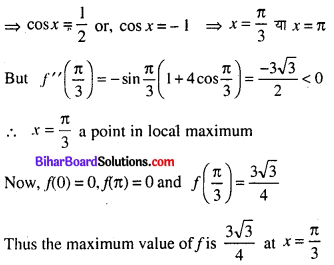Question 28.
Find the area of the parabola’s enclosed by the region y2 = 4ax and x2 = 4ay, where a
Given parabola’s y2 = 4ax …(1)
and x2 = 4ay …(2)
On solving (1) and (2), we get point of intersection So, substituting the value of x from (1) in (2), we get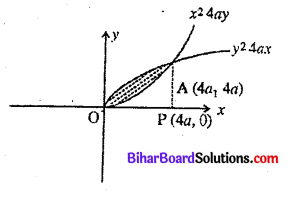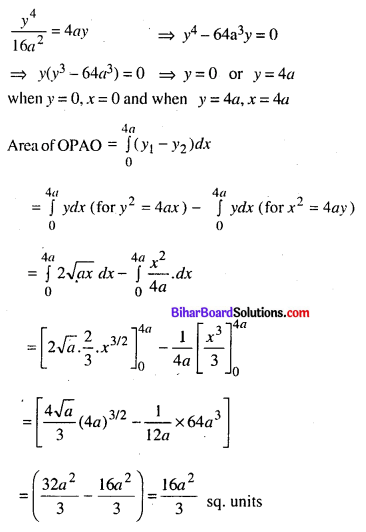Question 29.
Find the value at $$\int_{0}^{\pi / 4} \log (1+\tan x) d x$$Question 30.
Find the vector equation of plane which pases through the points A(3, -5, -1); B(-1, 5,7) and parallel to the
vector 3î – ĵ + 7k̂
Let the position vector of given points A and B are (3î – 5ĵ – k̂) and -î + 5ĵ + 7k̂
Let P be any point on the plane with position vector x
xî + yĵ + zk̂
AP=(x — 3)î + (y + 5)ĵ + (z +1)k̂
and AB = -4î + 10ĵ+ 8k̂
Clearly AP, AB and 3î – ĵ + 7k̂ are coplanar . So the equation of plane is
$$\left|\begin{array}{ccc} x-3 & y+5 & z+1 \\ -4 & 10 & 8 \\ 3 & -1 & 7 \end{array}\right|=0$$
⇒(x – 3) (70 + 8) – (y + 5)(-28 – 24) + (z +1)(4 – 30)= 0
⇒ 78 (x-3) + 52(y + 5)-26(z + l) = 0
⇒ 78x – 234 + 52y + 260 – 26z – 26 = 0
⇒ 78x + 52y-26z = 0
⇒ 3x + 2y-z = 0
The vector equation of plane is r. (3î – 5ĵ – k̂) = 0

Question 31.
Prove that the lines $$\frac{x+3}{-3}=\frac{y-1}{1}=\frac{z-5}{5}$$ are $$\frac{x+1}{-1}=\frac{y-2}{2}=\frac{z-5}{5}$$ coplanar.
Here x1 = -3, y1 = 1, z1 = 5, a1 = -3, b1 = 1, c1 =5
and x2 = -1 y2= 2, z2 = 5,a2 = -1 b2 = 2, c2 = 5
Given lines are copalanar if=2(5 – 10) – 1 (-15 + 5) + 0(-6 + 1)
= 2 x (-5) + 10 + 0 = 0
Hence the given lines are coplanar.Question 32.
Using LPP method, maximize Z = 10x + 6y subject to
constraints 3x +y ≤ 12,2x + 5y ≤ 34, x ≥ 0,y ≥ 0.
Maximize objeftive function Z = 10x + 6y
Subject to constraints
3x + y≤ 12 ⇒ 3x + y = 12 -..(1)
2x + 5y ≤ 34 ⇒ 2x + 5y = 34 …(2)
x ≥ 0, y ≥ 0 ⇒ x = 0,y = 0 …(3)
First of all draw graph of equations (1) to (3) corTes ponds to the inequations, we see from figure, the feasible, region OAPD be form, which is bounded also.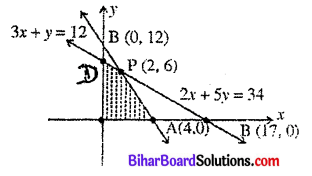The co-ordinate of the comer point of the feasible region, are 0(0, 0), A(4, 0), P(2, 6) and D(0, 34/5). Lastly, by using comer point method to find the maximum value of Z are as follows :It is obvious from the above table that maximum value of Z is 56 at the point (2,6). Thus the maximum value of Z is 56.Question 33.
A manufacturer produces nuts and bolts. It takes 1 hour of work on machine A and 3 hours on machine B to produce a package of nuts. It takes 3 hours on machine A and 1 hour on machine B to produce a package of bolts. He earns a profit of Rs. 17.50 per packages on nuts and Rs. 7.00 per package on bolts. How many package of each should be produced each day so as to maximise his profit, if he operates his machines for at the most 12 hours a day ?
Let x nuts and y bolts are produced. We have the given data, as follows: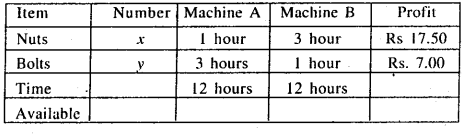Now, the time of machine A is used. = (x + 3y) hours Avilable time = 12 hours,
So, x + 3y ≤ 12 ⇒ x + 3y = 12 …(1)
The time of machine B is used = (3x + y) hours
Available time = 12 hours, So 3x + y ≤ 12
⇒ 3x + y = 12 …. (2)
Profit function Z = 17.5x + 7y is objective function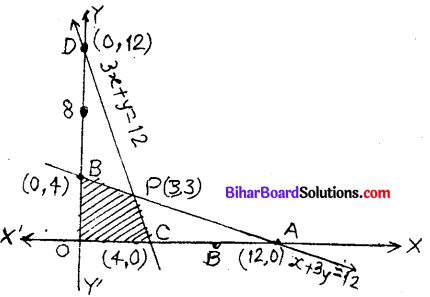Also, non-negative constraints
x ≥ 0, y ≥ 0 ⇒ x = 0, y = 0 …(3)
Now, linear equation (1) corresponds to inequation passes through the points A( 12, 0) and B(0, 4)
Putting x = 0, y = 0 in x + 3y ≤ 12,
we get 0 ≤ 12, which is true
So x + 3y ≤ 12 lies on and below AB The linear equation (2) corresponds to inequation passes through C(4, 0) and D(0. 12)
Putting x = 0, v = 0 in 3x + y ≤ 12,
we get 0 ≤ 12 which is true
So, 3x + y ≤ 12 lies on and below CD.
x ≥ 0 is the region which lies on and to the right on y-axis
y ≥ 0 is the region which lies on and above the x-axis
The line AB and CD intersects at the point P(3,3) Clearly it is obvious from figure the region OCPB is feasible region and is bounded. Lastly, calculate the value of Z= 17.5x + 7y as follows.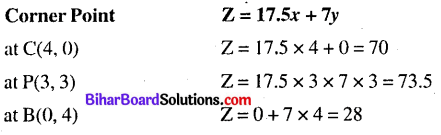From above table, it is clear that the maximum profit is Rs. 73.50 when 3 nuts and 3 bolts packages are produced.

error: Content is protected !!